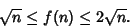## RAT-Free Set

A RAT-free (right angle triangle-free'') set is a set of points, no three of which determine a Right Triangle. Letbe the largest integer such that a RAT-free subset of sizeis guaranteed to be contained in any set ofcoplanar points. Then the functionis bounded byReferences

Abbott, H. L. On a Conjecture of Erdös and Silverman in Combinatorial Geometry.'' J. Combin. Th. A 29, 380-381, 1980.

Chan, W. K. On the Largest RAT-FREE Subset of a Finite Set of Points.'' Pi Mu Epsilon 8, 357-367, 1987.

Honsberger, R. More Mathematical Morsels. Washington, DC: Math. Assoc. Amer., pp. 250-251, 1991.

Seidenberg, A. A Simple Proof of a Theorem of Erdös and Szekeres.'' J. London Math. Soc. 34, 352, 1959.